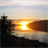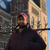# App Development

Announcements
Skip the ticket, Chat with Qlik Support instead for instant assistance.
cancel
Showing results for
Did you mean:Contributor II

## Max and aggregate

Hi all,

I want to calculate max of sum like example below

dateIDStickerAmount
1T1A100
1T1B200
1T1C300
2T1A200
2T1B100
2T1C400
1T2D2
1T2E3
1T2F5
2T2D8
2T2E7
2T2F1

I want the result is :T1 =  200+100+400=700

T2=8+7+1=16

this means Sum Amount based on each date, and choose Max number for each ID T.

IDAmount
T1700
T215

I used to a set analysis: max(aggr(sum(Amount),Date))

But it doesnt work

1 Solution

Accepted SolutionsPartner - Creator III

Hi, this works for me:

Max(Aggr(Sum(Amount),date, ID))

5 RepliesChampion

Hi,

for T1, if you need max(amount) for each date, it will be:

T1= 300 (for date 1) + 400 (for date 2) = 700

for T2:

T2= 5 (for date 1) + 8 (for date 2) = 13Partner - Creator III

Hi, this works for me:

Max(Aggr(Sum(Amount),date, ID))Champion

try this expression:

=sum(aggr(max(Amount),ID,date))Contributor II
Author

It is right, awesome. Thanks so muchPartner - Creator III

Do you mind marking it as correct?Tags
Community Browser